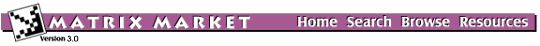## BCSSTK33: BCS Structural Engineering Matrices (large eigenvalue problems)Statics module of a pin boss (part of an automobile steering mechanism)

### from set BCSSTRUC5, from the Harwell-Boeing Collection

[Download] [Visualizations] [Matrix Statistics] [Set Information]

Stiffness matrix

## Download as

Help: My browser can't read the compressed data files. What now?

## Visualizations

Click on image to get an enlarged version. Click on label to get an explanation.

## Matrix Statistics

```
* * * * * * * * * * * * * * * * * * * * * * * * * * * * * * * * * * * * * *
*                           Matrix Statistics                             *
*              Generated by Yousef Saad's SPARSKIT Library                *
* * * * * * * * * * * * * * * * * * * * * * * * * * * * * * * * * * * * * *
* * * * * * * * * * * * * * * * * * * * * * * * * * * * * * * * * * * * * *
* TIFFNESS MATRIX FOR PIN BOSS (AUTO STEERING COMPONENT), SOLID ELEMENTS  *
*                    Key = BCSSTK33 , Type = PSA                          *
* No values provided - Information on pattern only                        *
* * * * * * * * * * * * * * * * * * * * * * * * * * * * * * * * * * * * * *
*  Dimension N                                      =       8738  *
*  Number of nonzero elements                       =     300321  *
*  Average number of nonzero elements/Column        =    34.3695  *
*  Standard deviation for above average             =     9.2854  *
*  Nonzero elements in strict lower part            =     291583  *
*  Nonzero elements in strict upper part            =     291583  *
*  Nonzero elements in main diagonal                =       8738  *
*  Weight of longest column                         =        142  *
*  Weight of shortest column                        =         19  *
*  Matching elements in symmetry                    =          0  *
*  Relative Symmetry Match (symmetry=1)             = NaN         *
*  Average distance of a(i,j)  from diag.           =  0.259E+03  *
*  Standard deviation for above average             =  0.200E+03  *
*-----------------------------------------------------------------*
*  Lower bandwidth  (max: i-j, a(i,j) .ne. 0)       =          0  *
*  Upper bandwidth  (max: j-i, a(i,j) .ne. 0)       =        932  *
*  Maximum Bandwidth                                =        933  *
*  Average Bandwidth                                =  0.406E+03  *
*  Number of nonzeros in skyline storage            =    3580133  *
*  90% of matrix is in the band of width            =        905  *
*  80% of matrix is in the band of width            =        851  *
*  The total number of nonvoid diagonals is         =        889  *
*  The 10 most important diagonals are (offsets)    :             *
*     0     1     2     3   421     4   422   420   423   419     *
*  The accumulated percentages they represent are   :             *
*   2.9   5.6   8.1  10.5  12.3  14.1  15.9  17.6  19.1  20.7     *
*-----------------------------------------------------------------*
*  The matrix does not have a block structure                     *
*-----------------------------------------------------------------*

```

## Set Information

Set BCSSTRUC5
Source: Boeing Computer Services
Discipline:Structural engineering
Accession:Spring 1986

The Matrix Market is a service of the Mathematical and Computational Sciences Division / Information Technology Laboratory / National Institute of Standards and Technology.

[ Home ] [ Search ] [ Browse ] [ Resources ]

Last change in this page: Wed Sep 22 13:33:28 US/Eastern 2004 [Comments: ]